Take the Last leap| Aakash BYJU'S JEE (Adv) '23 Mock Test on 28th May Take the Last leap| Aakash BYJU'S JEE (Adv) '23 Mock Test on 28th May

# JEE Main 2022 June 28 – Shift 2 Physics Question Paper with Solutions

Students can instantly download the JEE Main 2022 June 28 – Shift 2 Physics Question Paper from this page. The answer key is included with the question paper, and it has been meticulously created by our subject matter experts. It will help students grasp concepts more easily, and have a better understanding of the topics included in the question paper. The answers will also be simple for students to understand, and they will have a deeper understanding of how the Physics paper was set, the types of questions that were asked, the scoring system, the length of the question paper, and other factors.

## JEE Main 2022 June 28th Physics Shift 2 Question Paper and Solutions

#### SECTION – A

Multiple Choice Questions: This section contains 20 multiple choice questions. Each question has 4 choices (1), (2), (3) and (4), out of which ONLY ONE is correct.

1. Velocity (v) and acceleration (a) in two systems of units 1 and 2 are related as

$$\begin{array}{l}v_{2}=\frac{n}{m^{2}} v_{1}\ \text{and}\ a_{2}=\frac{a_{1}}{m n}\end{array}$$
respectively. Here m and n are constants. The relations for distance and time in two systems respectively are :

$$\begin{array}{l}(A)\ \frac{n^{3}}{m^{3}} L_{1}=L_{2}\ and\ \frac{n^{2}}{m} T_{1}=T_{2}\end{array}$$
$$\begin{array}{l}(B)\ L_{1}=\frac{n^{4}}{m^{2}} L_{2}\ and\ T_{1}=\frac{n^{2}}{m} T_{2}\end{array}$$
$$\begin{array}{l}(C)\ L_{1}=\frac{n^{2}}{m} L_{2}\ and\ T_{1}=\frac{n^{4}}{m^{2}} T_{2}\end{array}$$
$$\begin{array}{l}(D)\ \frac{n^{2}}{m} L_{1}=L_{2}\ and\ \frac{n^{4}}{m^{2}} T_{1}=T_{2}\end{array}$$

Sol.

$$\begin{array}{l}[L]=\frac{\left[v^{2}\right]}{[a]}\end{array}$$

so

$$\begin{array}{l}\frac{\left[v_{2}\right]^{2}}{\left[a_{2}\right]}=\frac{\left[\frac{n}{m^{2}} v_{1}\right]^{2}}{\left[\frac{a_{1}}{m n}\right]}\end{array}$$
$$\begin{array}{l}\frac{\left[v_{2}\right]^{2}}{\left[a_{2}\right]}=\frac{n^{3}}{m^{3}}\frac{\left[v_{1}\right]^{2}}{\left[a_{1}\right]}\end{array}$$
$$\begin{array}{l}\text { or }\left[L_{2}\right]=\frac{n^{3}}{m^{3}}\left[L_{1}\right]\end{array}$$

Similarly

$$\begin{array}{l}{[T]=\frac{[\mathrm{v}]}{[a]}}\end{array}$$

So,

$$\begin{array}{l}\left[T_{2}\right]=\frac{n^{2}}{m}\left[T_{1}\right]\end{array}$$

2. A ball is spun with angular acceleration α = 6t2 – 2t, where t is in second and α is in rads–2. At t = 0, the ball has angular velocity of 10 rads–1 and angular position of 4 rad. The most appropriate expression for the angular position of the ball is :

$$\begin{array}{l}(A)\ \frac{3}{4} t^{4}-t^{2}+10 t\end{array}$$
$$\begin{array}{l}(B)\ \frac{t^{4}}{2}-\frac{t^{3}}{3}+10 t+4\end{array}$$
$$\begin{array}{l}(C)\ \frac{2 t^{4}}{3}-\frac{t^{3}}{6}+10 t+12\end{array}$$
$$\begin{array}{l}(D)\ 2 t^{4}-\frac{t^{3}}{2}+5 t+4\end{array}$$

Sol.

$$\begin{array}{l}\alpha=\frac{d \omega}{d t}=6 t^{2}-2 t\end{array}$$
$$\begin{array}{l}\int_{0}^{\omega} d \omega=\int_{0}^{t}\left(6 t^{2}-2 t\right) d t\end{array}$$

so ω = 2t3t2 + 10

and

$$\begin{array}{l}\frac{d \theta}{d t}=2 t^{3}-t^{2}+10\end{array}$$

so

$$\begin{array}{l}\int_{4}^{\theta} d \theta=\int_{0}^{t}\left(2 t^{3}-t^{2}+10\right) d t\end{array}$$
$$\begin{array}{l}\theta=\frac{t^{4}}{2}-\frac{t^{3}}{3}+10 t+4\end{array}$$

3. A block of mass 2 kg moving on a horizontal surface with speed of 4 ms–1 enters a rough surface ranging from x = 0.5 m to x = 1.5 m. The retarding force in this range of rough surface is related to distance by F = –kx where k = 12 Nm–1. The speed of the block as it just crosses the rough surface will be :

(A) Zero

(B) 1.5 ms–1

(C) 2.0 ms–1

(D) 2.5 ms–1

Sol. F = –12x

$$\begin{array}{l}m v \frac{d v}{d x}=-12\end{array}$$
$$\begin{array}{l}\int_{4}^{v} v d v=-6 \int_{0.5}^{1.5} x d x\end{array}$$
(m = 2 kg)

$$\begin{array}{l}\frac{v^{2}-16}{2}=-6\left[\frac{1.5^{2}-0.5^{2}}{2}\right]\end{array}$$
$$\begin{array}{l}\frac{v^{2}-16}{2}=-6\end{array}$$

v = 2 m/sec

4. A √(34) m long ladder weighing 10 kg leans on a frictionless wall. Its feet rest on the floor 3 m away from the wall as shown in the figure. If Ff and Fw are the reaction forces of the floor and the wall, then ratio of Fw/Ff will be:

(Use g = 10 m/s2)$$\begin{array}{l}(A)\ \frac{6}{\sqrt{110}}\end{array}$$
$$\begin{array}{l}(B)\ \frac{3}{\sqrt{113}}\end{array}$$
$$\begin{array}{l}(C)\ \frac{3}{\sqrt{109}}\end{array}$$
$$\begin{array}{l}(D)\ \frac{2}{\sqrt{109}}\end{array}$$

Sol.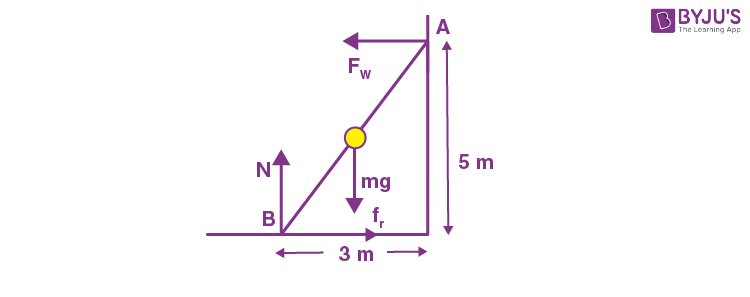Taking torque from B

$$\begin{array}{l}F_{w} \times 5=\frac{3}{2} m g\end{array}$$
$$\begin{array}{l}\Rightarrow \quad F_{w}=\frac{3}{10} \times 10 \times 10\end{array}$$

= 30 N

N = mg = 100 N

and fr = Fw = 30 N

so

$$\begin{array}{l}F_{f}=\sqrt{N^{2}+f_{r}^{2}}=\sqrt{10900}=10 \sqrt{109}\ N\end{array}$$

so

$$\begin{array}{l}\frac{F_{w}}{F_{f}}=\frac{3}{\sqrt{109}}\end{array}$$

5. Water falls from a 40 m high dam at the rate of 9 × 104 kg per hour. Fifty percentage of gravitational potential energy can be converted into electrical energy. Using this hydro electric energy number of 100 W lamps, that can be lit, is :

(Take g = 10 ms–2)

(A) 25

(B) 50

(C) 100

(D) 18

Sol. Total gravitational PE of water per second

$$\begin{array}{l}=\frac{mgh}{T}\end{array}$$
$$\begin{array}{l}=\frac{9 \times 10^{4} \times 10 \times 40}{3600}=10^{4} \mathrm{~J} / \mathrm{sec}\end{array}$$

50% of this energy can be converted into electrical energy so total electrical energy

$$\begin{array}{l}=\frac{10^{4}}{2}=5000 \mathrm{~W}\end{array}$$

So total bulbs lit can be

$$\begin{array}{l}=\frac{5000 \mathrm{~W}}{100 \mathrm{~W}}\end{array}$$
= 50 bulbs

6. Two objects of equal masses placed at certain distance from each other attracts each other with a force of F. If one-third mass of one object is transferred to the other object, then the new force will be

$$\begin{array}{l}(A)\ \frac{2}{9} F\end{array}$$
$$\begin{array}{l}(B)\ \frac{16}{9} F\end{array}$$
$$\begin{array}{l}(C)\ \frac{8}{9} F\end{array}$$

(D) F

Sol.Let the masses are m and distance between them is l, then

$$\begin{array}{l}F=\frac{G m^{2}}{I^{2}}\end{array}$$

When 1/3rd mass is transferred to the other then masses will be 4m/3 and 2m/3. So new force will be

$$\begin{array}{l}F^{\prime}=\frac{G \frac{4 m}{3} \times \frac{2 m}{3}}{I^{2}}=\frac{8}{9} \frac{G m^{2}}{I^{2}}=\frac{8}{9} F\end{array}$$

7. A water drop of radius 1 μm falls in a situation where the effect of buoyant force is negligible. Co-efficient of viscosity of air is 1.8 × 10–5 Nsm–2 and its density is negligible as compared to that of water (106 gm–3). Terminal velocity of the water drop is

(Take acceleration due to gravity = 10 ms–2)

(A) 145.4 × 10–6 ms–1

(B) 118.0 × 10–6 ms–1

(C) 132.6 × 10–6 ms–1

(D) 123.4 × 10–6 ms–1

Sol.

$$\begin{array}{l}6 \pi \eta r v=m g\end{array}$$
$$\begin{array}{l}6 \pi \eta r v=\frac{4}{3} \pi r^{3} \rho g\end{array}$$

or

$$\begin{array}{l}v=\frac{2}{9} \frac{\rho r^{2} g}{\eta}=\frac{2}{9} \times \frac{10^{3} \times\left(10^{-6}\right)^{2} \times 10}{1.8 \times 10^{-5}}\end{array}$$

= 123.4×10–6 m/s

8. A sample of an ideal gas is taken through the cyclic process ABCA as shown in figure. It absorbs, 40 J of heat during the part AB, no heat during BC and rejects 60 J of heat during CA. A work of 50 J is done on the gas during the part BC. The internal energy of the gas at A is 1560 J. The work done by the gas during the part CA is: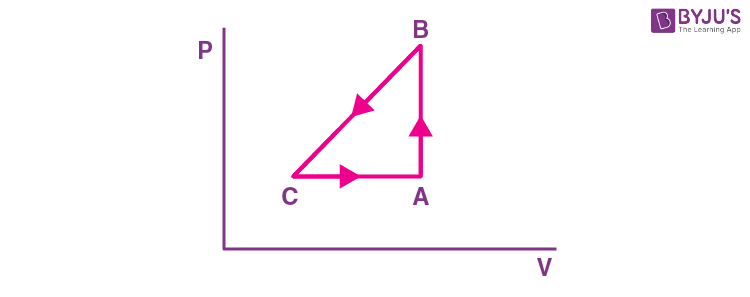(A) 20 J

(B) 30 J

(C) –30 J

(D) –60 J

Sol. ΔUAB = 40 J as process is isochoric.

ΔUBC + WBC = 0

ΔUBC = +50 (WBC = –50 J)

UC = UA + ΔUAB + ΔUBC = 1650

For CA process,

QCA = – 60 J

ΔUCA + WCA = –60

90 + WCA = –60

WCA = +30 J

The graph given is inconsistent with the statement BC may be adiabatic and CA cannot be like isobaric as shown, as increasing volume while rejecting heat at same time.

9. What will be the effect on the root mean square velocity of oxygen molecules if the temperature is doubled and oxygen molecule dissociates into atomic oxygen?

(A) The velocity of atomic oxygen remains same

(B) The velocity of atomic oxygen doubles

(C) The velocity of atomic oxygen becomes half

(D) The velocity of atomic oxygen becomes four times

Sol. As

$$\begin{array}{l}v_{\mathrm{rms}}=\sqrt{\frac{3 R T}{M_{0}}}\end{array}$$

T is doubled and oxygen molecule is dissociated into atomic oxygen molar mass is halved.

So,

$$\begin{array}{l}v_{\mathrm{rms}}^{\prime}=\sqrt{\frac{3 R \times 2 T_{0}}{M_{0} / 2}}=2 v_{\mathrm{rms}}\end{array}$$

So velocity of atomic oxygen is doubled.

10. Two point charges A and B of magnitude +8 × 10–6 C and –8 × 10–6 C respectively are placed at a distance d apart. The electric field at the middle point O between the charges is 6.4 × 104 NC–1. The distance ‘d’ between the point charges A and B is:

(A) 2.0 m

(B) 3.0 m

(C) 1.0 m

(D) 4.0 m

Sol.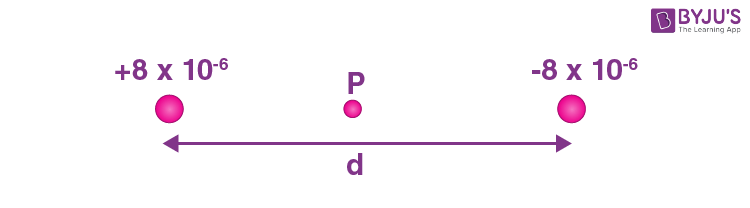Electric field at P will be

$$\begin{array}{l}E=\frac{k q}{(d / 2)^{2}} \times 2=\frac{8 k q}{d^{2}}\end{array}$$

So,

$$\begin{array}{l}\frac{8 \times 9 \times 10^{9} \times 8 \times 10^{-6}}{d^{2}}=6.4 \times 10^{4}\end{array}$$

So, d = 3 m

11. Resistance of the wire is measured as 2 Ω and 3 Ω at 10°C and 30°C respectively. Temperature co-efficient of resistance of the material of the wire is:

(A) 0.033°C–1

(B) –0.033°C–1

(C) 0.011°C–1

(D) 0.055°C–1

Sol. R10 = 2 = R0(1 + α × 10)

R30 = 3 = R0(1 + α × 30)

On solving

α = 0.033/°C

12. The space inside a straight current carrying solenoid is filled with a magnetic material having magnetic susceptibility equal to 1.2 × 10–5. What is fractional increase in the magnetic field inside solenoid with respect to air as medium inside the solenoid?

(A) 1.2 × 10–5

(B) 1.2 × 10–3

(C) 1.8 × 10–3

(D) 2.4 × 10–5

Sol.

$$\begin{array}{l}\overrightarrow{B’}=\mu_0(1 + X)ni\ \text{in the material}\end{array}$$
$$\begin{array}{l}\overrightarrow{B}=\mu_0ni\ \text{without material}\end{array}$$

So fractional increase is

$$\begin{array}{l}\frac{B^{\prime}-B}{B}=X=1.2 \times 10^{-5}\end{array}$$

13. Two parallel, long wires are kept 0.20 m apart in vacuum, each carrying current of x A in the same direction. If the force of attraction per meter of each wire is 2 × 10–6 N, then the value of x is approximately:

(A) 1

(B) 2.4

(C) 1.4

(D) 2

Sol.

$$\begin{array}{l}\frac{d F}{d l}=2 \times 10^{-6} \mathrm{~N} / \mathrm{m}=\frac{\mu_{0} i_{1} i_{2}}{2 \pi d}\end{array}$$
$$\begin{array}{l}2 \times 10^{-6}=\frac{2 \times 10^{-7} \times x^{2}}{0.2}\end{array}$$
$$\begin{array}{l}x=\sqrt{2} \simeq 1.4\end{array}$$

14. A coil is placed in a time varying magnetic field. If the number of turns in the coil were to be halved and the radius of wire doubled, the electrical power dissipated due to the current induced in the coil would be:

(Assume the coil to be short circuited.)

(A) Halved

(C) The same

(D) Doubled

Sol. As number of turns are halved so length of wire is halved, and radius is doubled, then area will be 4 times the previous one if previous resistance is R then new resistance is R/8 and if previous emf is E then new emf will be E/2 so

$$\begin{array}{l}P_{i}=\frac{E^{2}}{R}\end{array}$$
$$\begin{array}{l}P_{f}=\frac{(E / 2)^{2}}{R / 8}=\frac{2 E^{2}}{R}=2 P_{i}\end{array}$$

As the answer key is changing students can challenge this question.

15. An EM wave propagating in x-direction has a wavelength of 8 mm. The electric field vibrating
y-direction has maximum magnitude of 60 Vm–1. Choose the correct equations for electric and magnetic field if the EM wave is propagating in vacuum:

(A)

$$\begin{array}{l}E_{y}=60 \sin \left[\frac{\pi}{4} \times 10^{3}\left(x-3 \times 10^{8} t\right)\right] \hat{j} \mathrm{Vm}^{-1}\end{array}$$
$$\begin{array}{l}B_{z}=2 \sin \left[\frac{\pi}{4} \times 10^{3}\left(x-3 \times 10^{8} t\right)\right] \hat{k} \mathrm{~T}\end{array}$$

(B)

$$\begin{array}{l}E_{y}=60 \sin \left[\frac{\pi}{4} \times 10^{3}\left(x-3 \times 10^{8} t\right)\right] \hat{j} \mathrm{Vm}^{-1}\end{array}$$
$$\begin{array}{l}B_{z}=2 \times 10^{-7} \sin \left[\frac{\pi}{4} \times 10^{3}\left(x-3 \times 10^{8} t\right)\right] \hat{k} \mathrm{~T}\end{array}$$

(C)

$$\begin{array}{l}E_{y}=2 \times 10^{-7} \sin \left[\frac{\pi}{4} \times 10^{3}\left(x-3 \times 10^{8} t\right)\right] \hat{j} \mathrm{Vm}^{-1}\end{array}$$
$$\begin{array}{l}B_{z}=60 \sin \left[\frac{\pi}{4} \times 10^{3}\left(x-3 \times 10^{8} t\right)\right] \hat{k}T\end{array}$$

(D)

$$\begin{array}{l}E_{y}=2 \times 10^{-7} \sin \left[\frac{\pi}{4} \times 10^{4}\left(x-4 \times 10^{8} t\right)\right] \hat{j} \mathrm{Vm}^{-1}\end{array}$$
$$\begin{array}{l}B_{z}=60 \sin \left[\frac{\pi}{4} \times 10^{4}\left(x-4 \times 10^{8} t\right)\right] \hat{k} T\end{array}$$

Sol. In first 3 options speed of light is 3 × 108 m/sec and in the fourth option it is 4 × 108 m/sec.

Using

E = CB

We can check the option is B.

16. In Young’s double slit experiment performed using a monochromatic light of wavelength λ, when a glass plate (μ = 1.5) of thickness xλ is introduced in the path of the one of the interfering beams, the intensity at the position where the central maximum occurred previously remains unchanged. The value of x will be:

(A) 3

(B) 2

(C) 1.5

(D) 0.5

Sol. For the intensity to remain same the position must be of a maxima so path difference must be nλ so

(1.5 – 1)xλ = nλ

x = 2n (n = 0, 1, 2 …)

So, value of x will be

x = 0, 2, 4, 6…

17. Let K1 and K2 be the maximum kinetic energies of photo-electrons emitted when two monochromatic beams of wavelength λ1 and λ2, respectively are incident on a metallic surface. If λ1 = 3λ2 then:

$$\begin{array}{l}(A)\ K_1 > \frac{K_2}{3} \end{array}$$
$$\begin{array}{l}(B)\ K_1 < \frac{K_2}{3} \end{array}$$
$$\begin{array}{l}(C)\ K_1 = \frac{K_2}{3} \end{array}$$
$$\begin{array}{l}(D)\ K_2 = \frac{K_1}{3} \end{array}$$

Sol.

$$\begin{array}{l}K_{1}=\frac{h c}{\lambda_{1}}-\phi=\frac{h c}{3 \lambda_{2}}-\phi….(i)\end{array}$$

and

$$\begin{array}{l}K_{2}=\frac{h c}{\lambda_{2}}-\phi….(ii)\end{array}$$

from (i) and (ii) we can say

3K1 = K2 – 2φ

$$\begin{array}{l}K_{1}<\frac{K_{2}}{3}\end{array}$$

18. Following statements related to radioactivity are given below:

(A) Radioactivity is a random and spontaneous process and is dependent on physical and chemical conditions.

(B) The number of un-decayed nuclei in the radioactive sample decays exponentially with time.

(C) Slope of the graph of loge (no. of undecayed nuclei) Vs. time represents the reciprocal of mean life time (τ).

(D) Product of decay constant (λ) and half-life time (T1/2) is not constant.

Choose the most appropriate answer from the options given below:

(A) (A) and (B) only

(B) (B) and (D) only

(C) (B) and (C) only

(D) (C) and (D) only

Sol. Radioactive decay is a random and spontaneous process it depends on unbalancing of nucleus.

N = N0e–λt …(B)

lnN = –λt + lnN0

So, slope = – λ …(C)

$$\begin{array}{l}t_{1/2} = \frac{ln 2}{\lambda} \end{array}$$

So t1/2 × λ = ln2 = Constant

19. In the given circuit the input voltage Vin is shown in figure. The cut-in voltage of pn junction diode
(D1 or D2) is 0.6 V. Which of the following output voltage (V0) waveform across the diode is correct?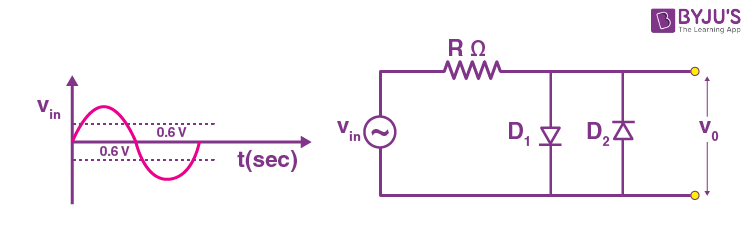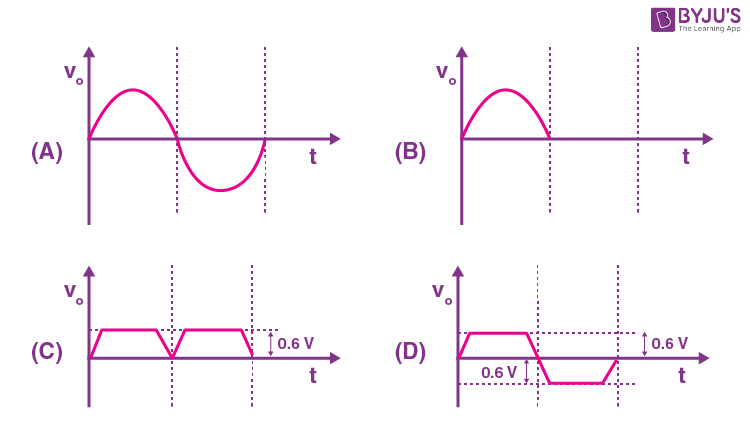Sol. Till

$$\begin{array}{l}\left| V \right| \le 0.6 ~\text{V}\end{array}$$
$$\begin{array}{l}\left| V_0 \right| = \left| V\right|\end{array}$$

So correct graph will be D.

20. Amplitude modulated wave is represented by VAM = 10[1 + 0.4 cos(2π × 104t] cos(2π × 107t). The total bandwidth of the amplitude modulated wave is:

(A) 10 kHz

(B) 20 MHz

(C) 20 kHz

(D) 10 MHz

Sol. Bandwidth = 2 × fm

= 2 × 104 Hz = 20 kHz

#### SECTION – B

Numerical Value Type Questions: This section contains 10 questions. In Section B, attempt any five questions out of 10. The answer to each question is a NUMERICAL VALUE. For each question, enter the correct numerical value (in decimal notation, truncated/rounded-off to the second decimal place; e.g. 06.25, 07.00, –00.33, –00.30, 30.27, –27.30) using the mouse and the on-screen virtual numeric keypad in the place designated to enter the answer.

1. A student in the laboratory measures thickness of a wire using screw gauge. The readings are 1.22 mm, 1.23 mm, 1.19 mm and 1.20 mm. The percentage error is

$$\begin{array}{l}\frac{x}{121}\%\end{array}$$
. The value of x is _________.

Sol.

$$\begin{array}{l}I_{\text {mean }}=\frac{1.22+1.23+1.19+1.20}{4}=1.21\end{array}$$
$$\begin{array}{l}\Delta I_{\text {mean }}=\frac{0.01+0.02+0.02+0.01}{4}=0.015\end{array}$$

So

$$\begin{array}{l}\%I= \frac{\Delta I_{\text {mean }}}{I_{\text {mean }}} \times 100=\frac{0.015}{1.21} \times 100\end{array}$$
$$\begin{array}{l}=\frac{150}{121} \%\end{array}$$

x = 150

2. A zener of breakdown voltage VZ = 8 V and maximum Zener current, IZM = 10 mA is subjected to an input voltage Vi = 10 V with series resistance R = 100 Ω. In the given circuit RL represents the variable load resistance. The ratio of maximum and minimum value of RL is __________.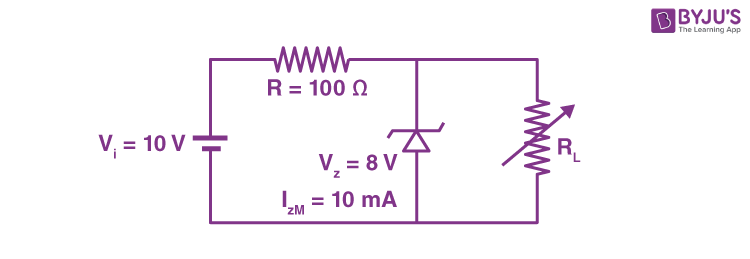Sol. Minimum value of RL for which the diode is shorted is

$$\begin{array}{l}\frac{R_{L}}{R_{L}+100} \times 10=8 \Rightarrow R_{L}=400 ~\Omega\end{array}$$

For maximum value of RL, current through diode is 10 mA.

So

$$\begin{array}{l}i_{R}=i_{R_{L}}+I_{Z M}\end{array}$$
$$\begin{array}{l}\frac{2}{100}=\frac{8}{R_{L}}+10 \times 10^{-3}\end{array}$$
$$\begin{array}{l}10 \times 10^{-3}=\frac{8}{R_{L}}\end{array}$$

RL = 800 Ω

So

$$\begin{array}{l}\frac{R_{L \max }}{R_{L \min }}=2\end{array}$$

3. In a Young’s double slit experiment, an angular width of the fringe is 0.35° on a screen placed at
2 m away for particular wavelength of 450 nm. The angular width of the fringe, when whole system is immersed in a medium of refractive index 7/5, is 1/α. The value of α is _________.

Sol. Angular fringe width

$$\begin{array}{l}\theta=\frac{\lambda}{D}\end{array}$$

So

$$\begin{array}{l}\frac{\theta_{1}}{\lambda_{1}}=\frac{\theta_{2}}{\lambda_{2}}\end{array}$$
$$\begin{array}{l}\theta_{2}=\frac{0.35^{\circ}}{450 \mathrm{~nm}} \times \frac{450 \mathrm{~nm}}{715}=0.25^{\circ}=\frac{1}{4}\end{array}$$

So α = 4

4. In the given circuit, the magnitude of VL and VC are twice that of VR. Given that f = 50 Hz, the inductance of the coil is 1/(Kπ) mH. The value of K is ________.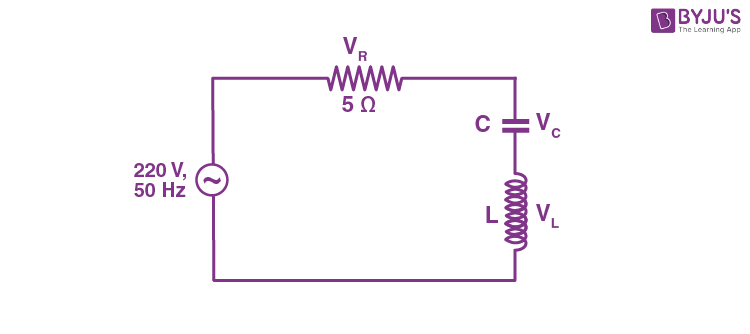Sol. VL = 2VR

So ωLi = 2 Ri

$$\begin{array}{l}\Rightarrow L = \frac{2 R}{\omega}=\frac{2 \times 5}{2 \pi \times 50}=\frac{1}{10 \pi} \mathrm{H}=\frac{100}{\pi} \mathrm{H}\end{array}$$

So

$$\begin{array}{l}k=\frac{1}{100} \simeq 0\end{array}$$

5. All resistances in figure are 1 Ω each. The value of current ‘I‘ is (a/5) A. The value of a is _______.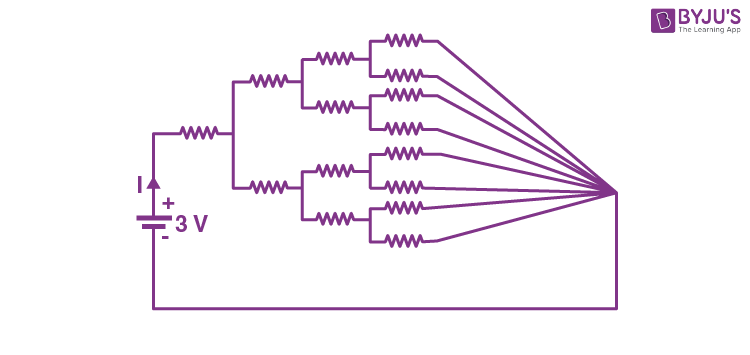Sol.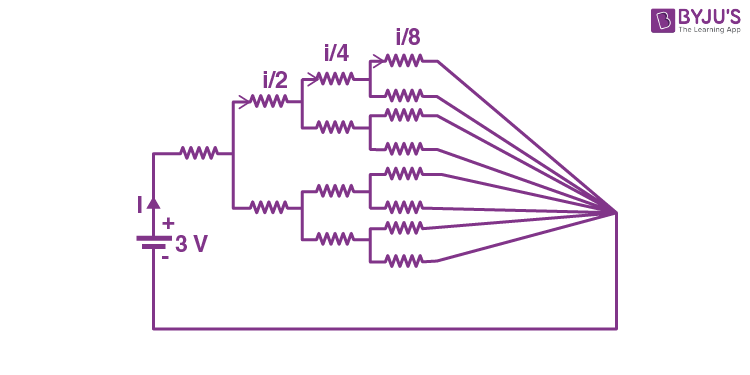Let the current is i

Using kirchhoff’s law

$$\begin{array}{l}i R+\frac{i}{2} R+\frac{i}{4} R+\frac{i}{8} R=3\end{array}$$
$$\begin{array}{l}i=\frac{3 \times 8}{15}=\frac{8}{5} \mathrm{~A}\end{array}$$

So a = 8

6. A capacitor C1 of capacitance 5 μF is charged to a potential of 30 V using a battery. The battery is then removed and the charged capacitor is connected to an uncharged capacitor C2 of capacitance 10 μF as shown in figure. When the switch is closed charge flows between the capacitors. At equilibrium, the charge on the capacitor C2 is ____ μC.Sol.Let the charge q is flown in the circuit.

So using Kirchoff’s law

$$\begin{array}{l}\frac{q}{10}=\frac{150-q}{5}\end{array}$$

q = 100 μC

7. A tuning fork of frequency 340 Hz resonates in the fundamental mode with an air column of length
125 cm in a cylindrical tube closed at one end. When water is slowly poured in it, the minimum height of water required for observing resonance once again is ____ cm.

(Velocity of sound in air is 340 ms–1)

Sol. Given

$$\begin{array}{l}340=\frac{n}{4 \times 125} v\end{array}$$

n = 5

So λ = 100 cm

So minimum height is

$$\begin{array}{l}\frac{\lambda}{2} = 50\ cm\end{array}$$

8. A liquid of density 750 kgm–3 flows smoothly through a horizontal pipe that tapers in
cross-sectional area from A1 = 1.2 × 10–2 m2 to

$$\begin{array}{l}A_2 = \frac{A_{1}}{2}\end{array}$$
. The pressure difference between the wide and narrow sections of the pipe is 4500 Pa. The rate of flow of liquid is _____ × 10–3 m3s–1.

Sol.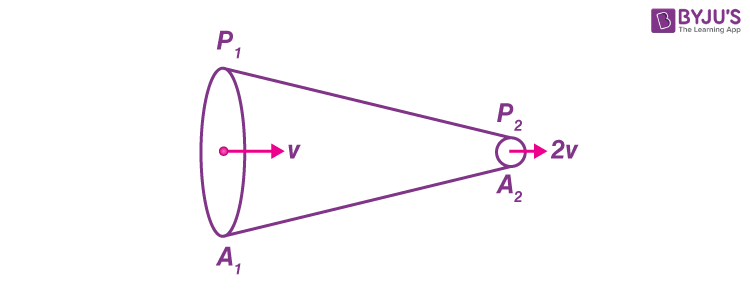Using Bernoulli’s equation

$$\begin{array}{l}P_{1}+\frac{1}{2} \rho v^{2}=P_{2}+\frac{1}{2} \rho 4 v^{2}\end{array}$$
$$\begin{array}{l}\frac{3}{2} \rho v^{2}=P_{1}-P_{2}\end{array}$$
$$\begin{array}{l}\Rightarrow v=\sqrt{\frac{2\left(P_{1}-P_{2}\right)}{3 \rho}}\end{array}$$
$$\begin{array}{l}=\sqrt{\frac{2 \times 4500}{3 \times 750}}=2 \mathrm{~m} / \mathrm{sec}\end{array}$$

So Q = A1v = 24 × 10–3 m3/sec

9. A uniform disc with mass M = 4 kg and radius R = 10 cm is mounted on a fixed horizontal axle as shown in figure. A block with mass m = 2 kg hangs from a massless cord that is wrapped around the rim of the disc. During the fall of the block, the cord does not slip and there is no friction at the axle. The tension in the cord is _____ N.

(Take g = 10 ms–2)Sol.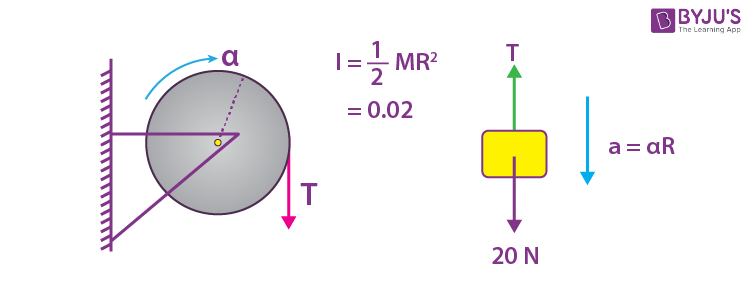20 – T = 2a

and 0.1 × T = 0.02 α

$$\begin{array}{l}= \frac{0.02a}{0.1}\end{array}$$

T = 2a

a = 5 m/sec2

So T = 10 N

10. A car covers AB distance with first one-third at velocity v1 ms–1, second one-third at v2 ms–1 and last one-third at v3 ms–1. If v3 = 3v1, v2 = 2v1 and v1 = 11 ms–1 then the average velocity of the car is ____ ms–1.Sol.

$$\begin{array}{l}v_{\text {mean }}=\frac{3 v_{1} v_{2} v_{3}}{v_{1} v_{2}+v_{2} v_{3}+v_{3} v_{1}}\end{array}$$
$$\begin{array}{l} = \frac{3 \times 11 \times 22 \times 33}{11 \times 22+22 \times 33+33 \times 11}\end{array}$$

= 18 m/sec

### Download PDF of JEE Main 2022 June 28 Shift 2 Physics Paper & Solutions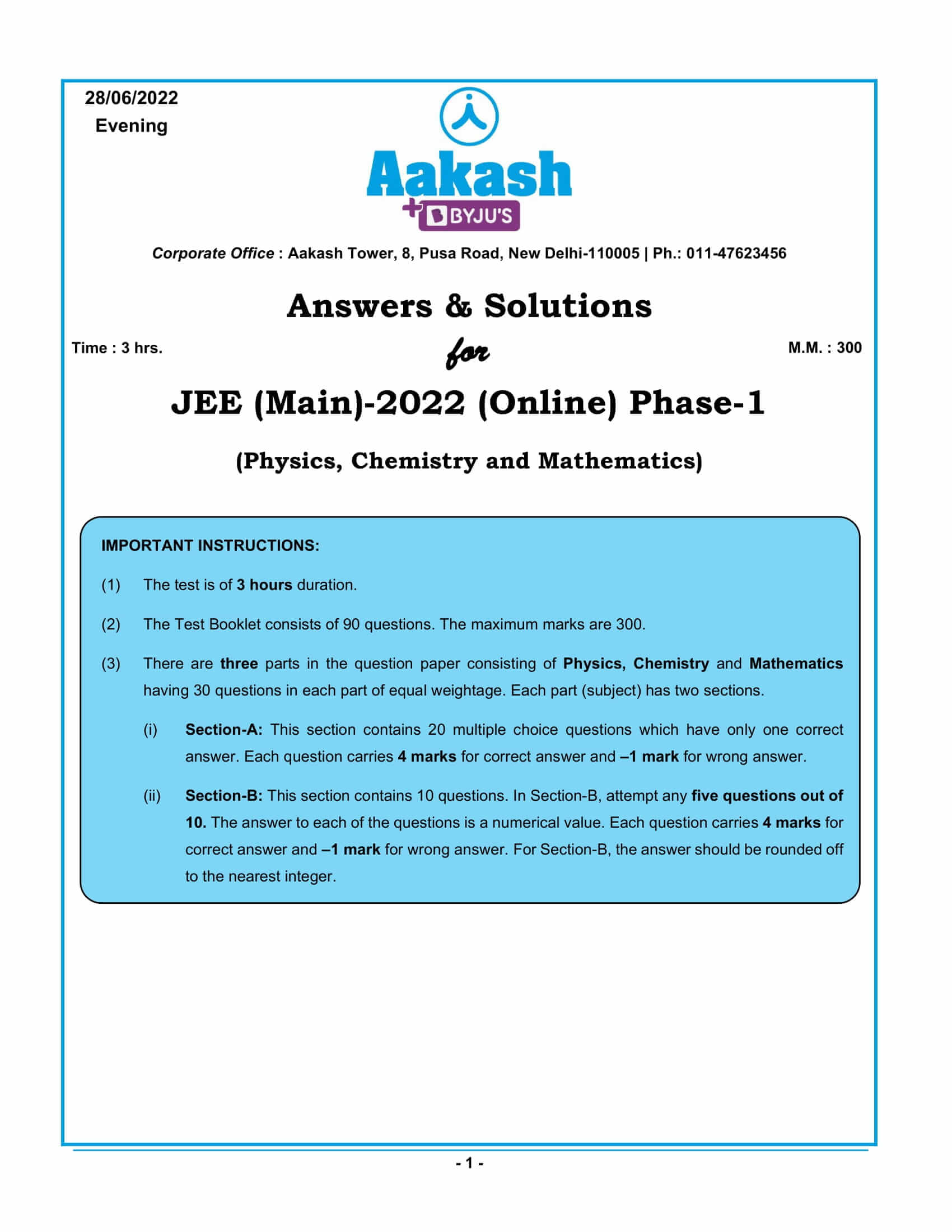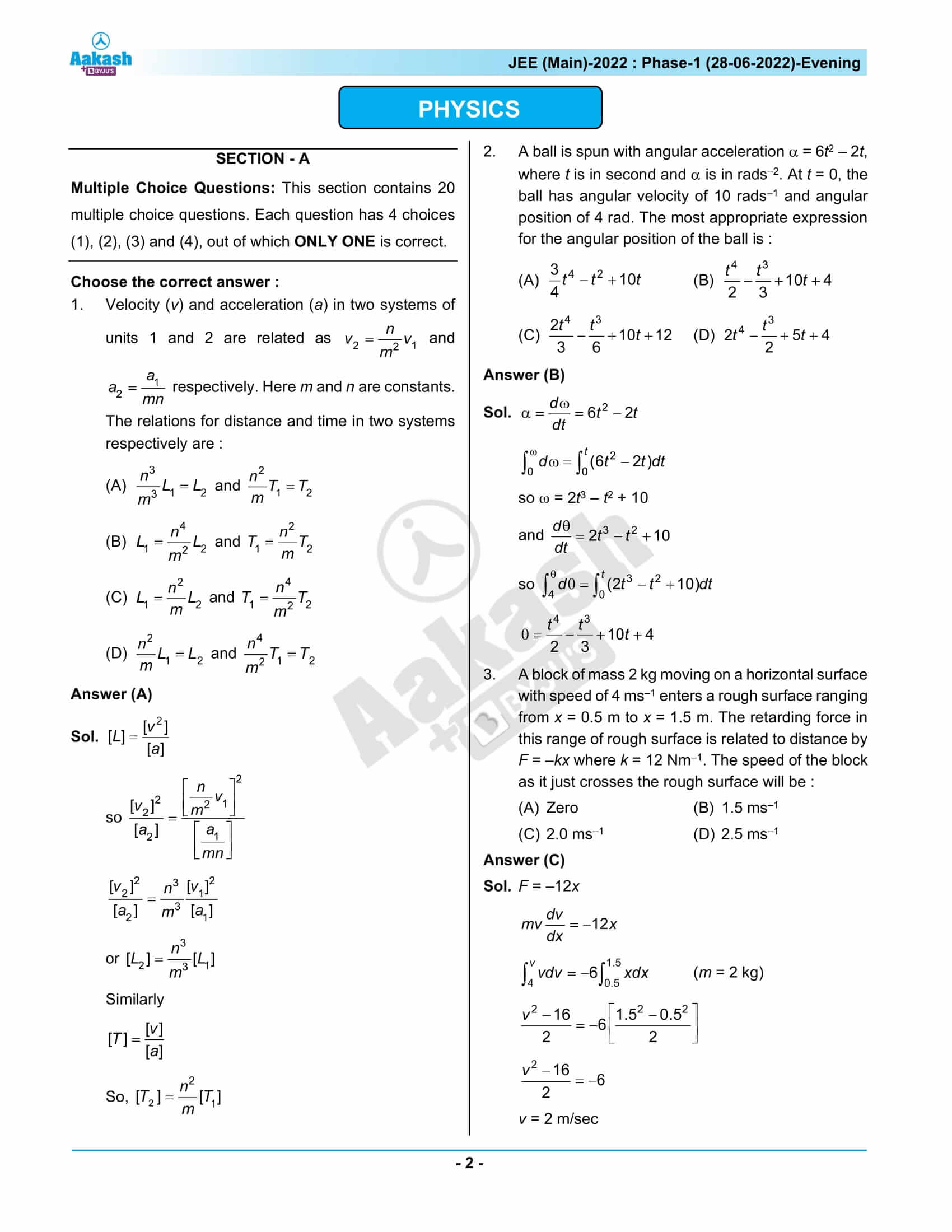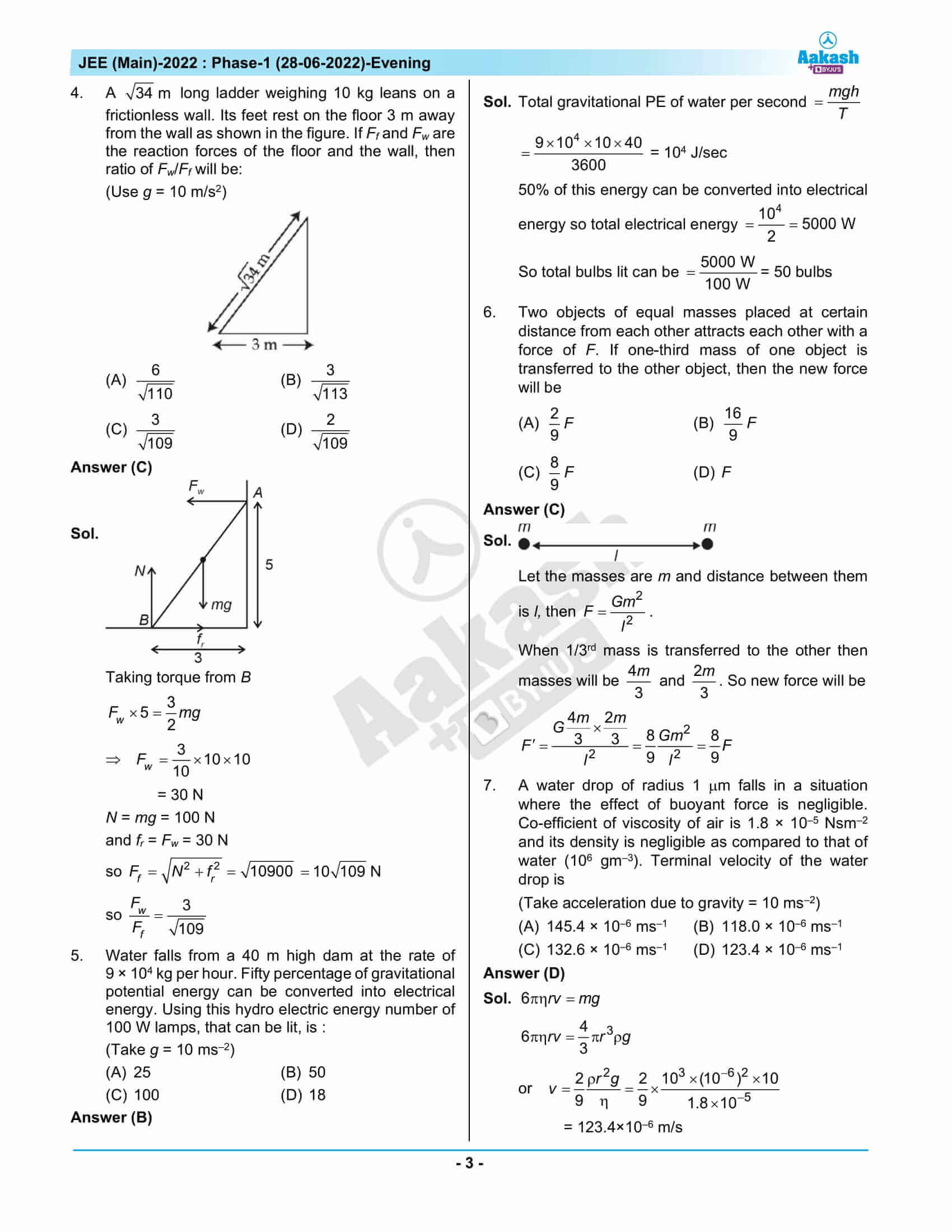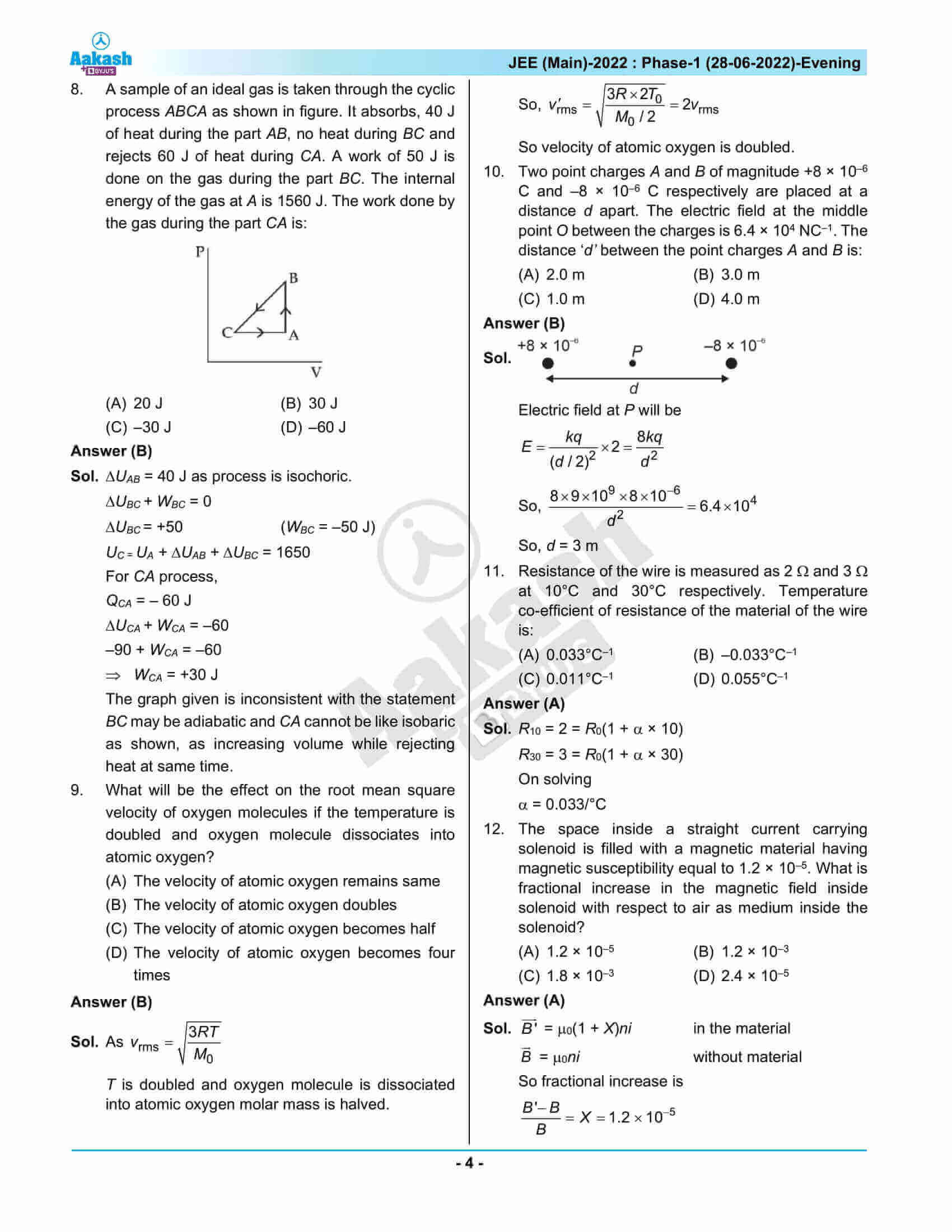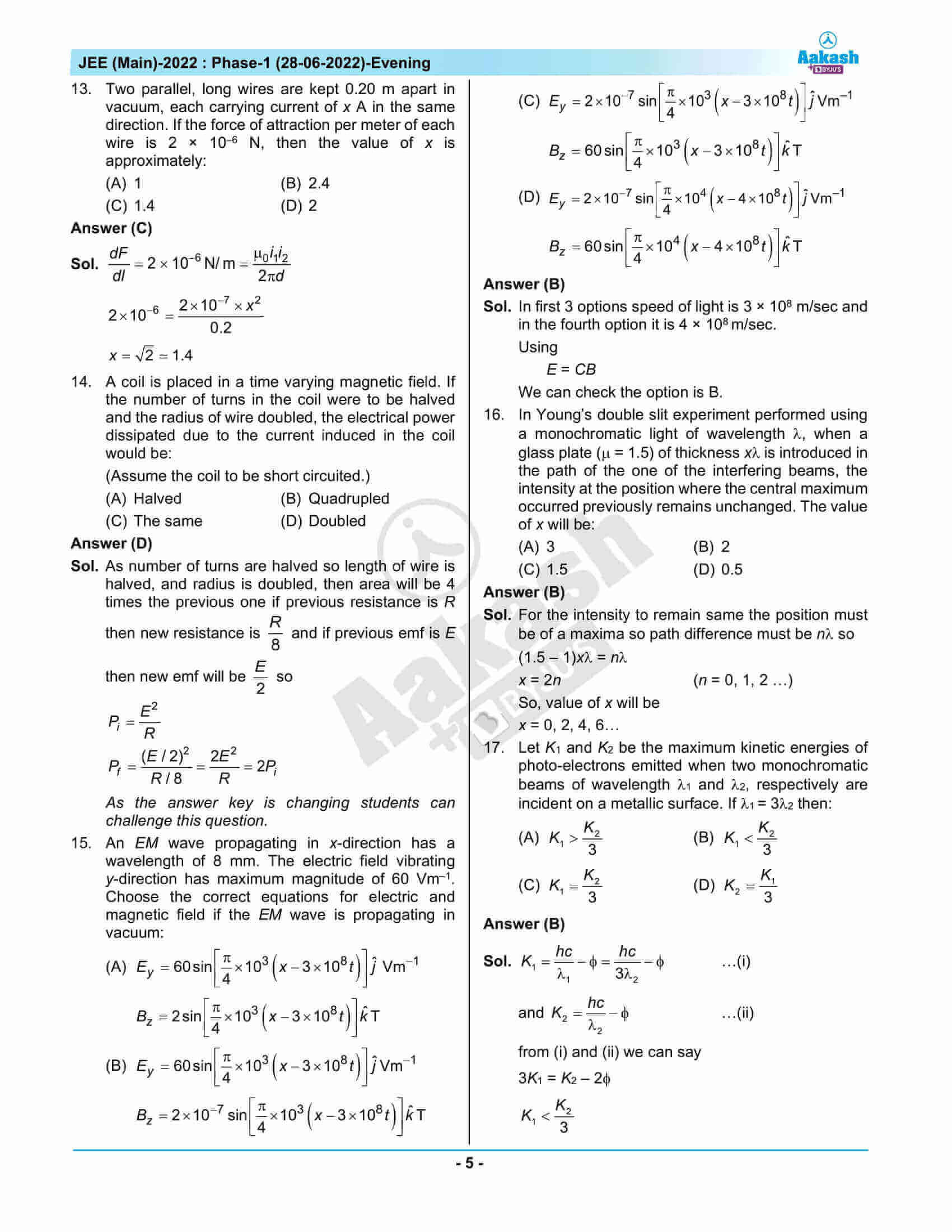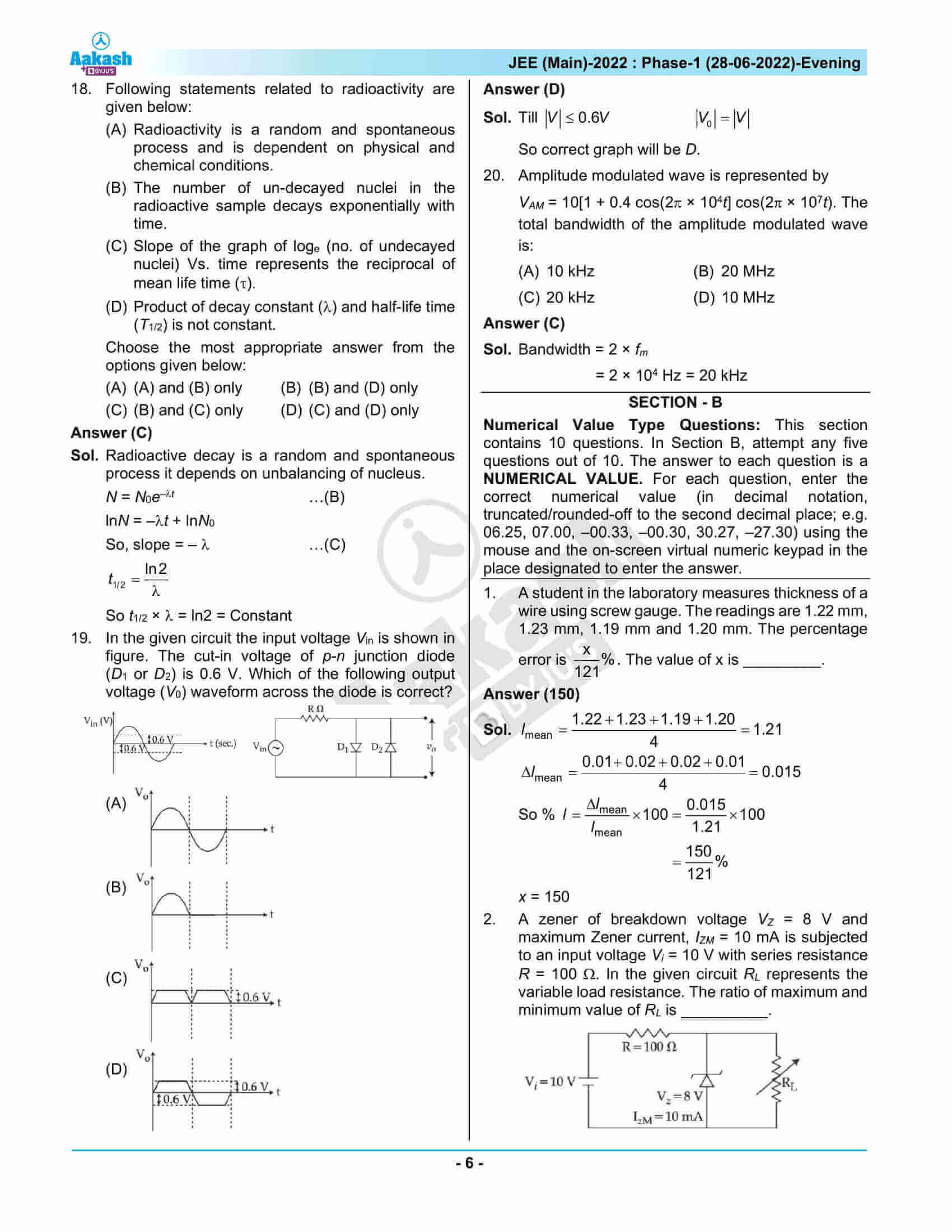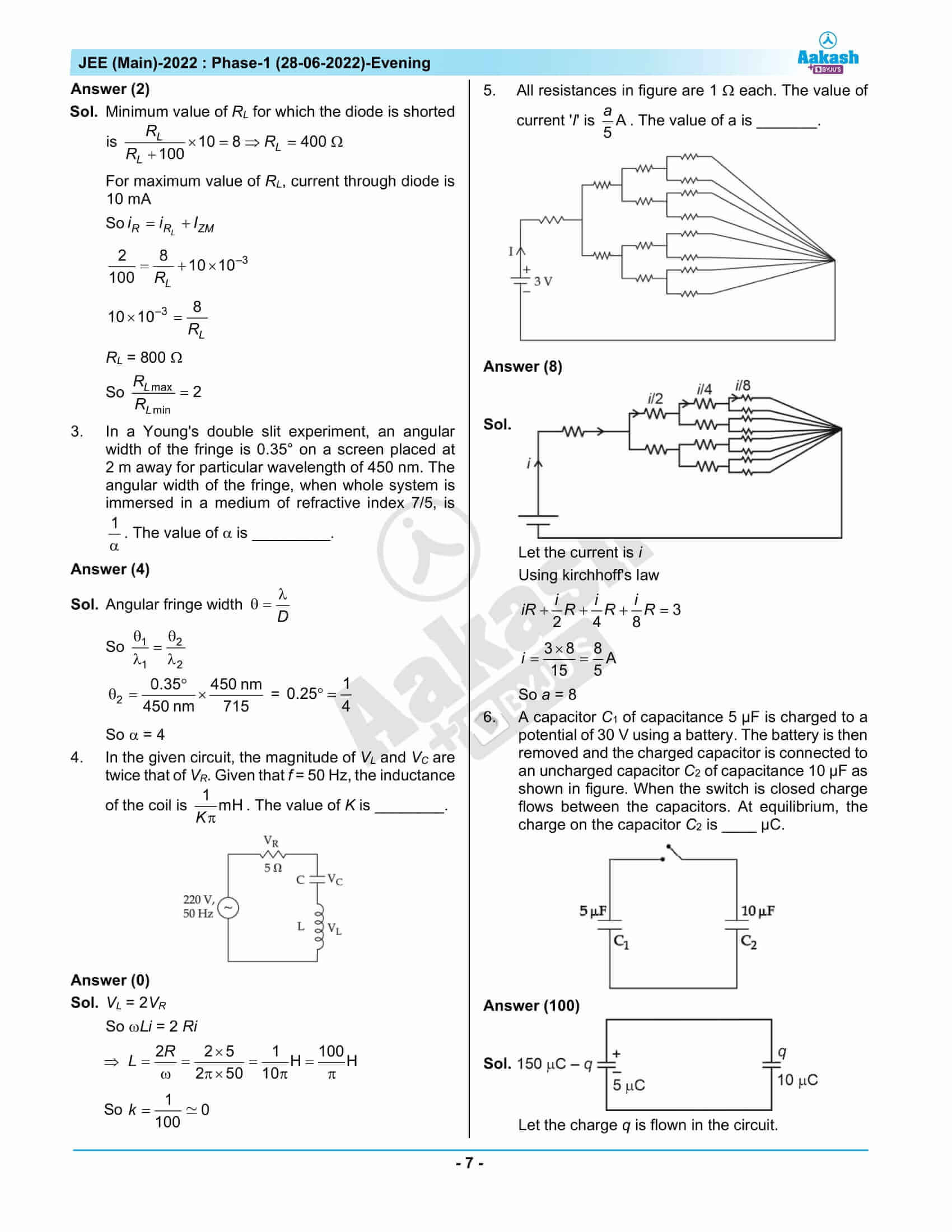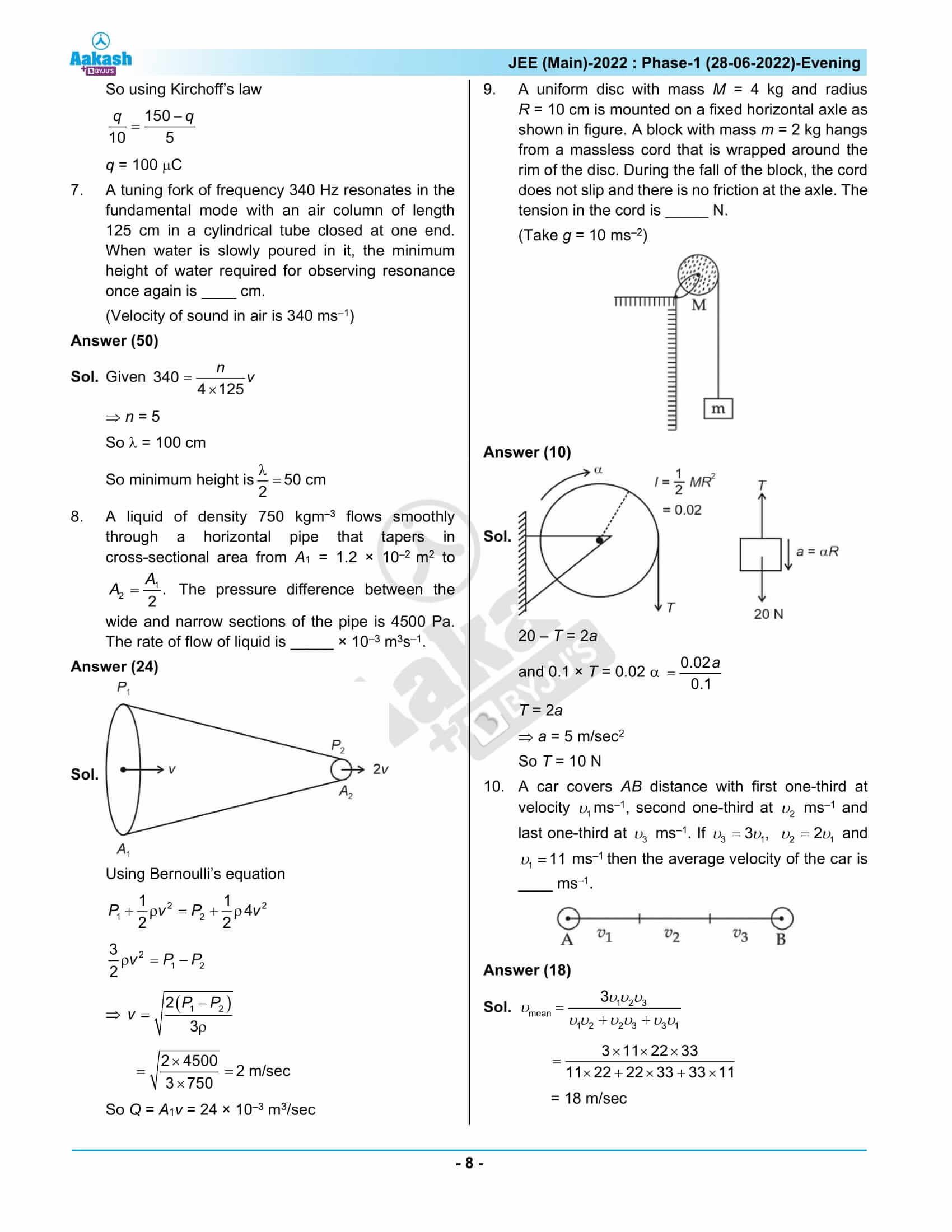## JEE Main 2022 Question Paper June 28th Shift 2 – Video Solutions

### JEE Main 2022 June 28 Shift 2 Question Paper – Physics Solutions### JEE Main 2022 June 28 Shift 2 Question Paper – Chemistry Solutions### JEE Main 2022 June 28 Shift 2 Question Paper – Maths Solutions## Frequently Asked Questions – FAQs

Q1

### How many questions were there in the physics section of the JEE Main 2022 June 28 shift 2 question paper?

JEE Main 2022 June 28 shift 2 Physics question paper had a total of 30 questions. Ten of these thirty questions were single-answer questions, and the remaining twenty were multiple choice. Only five of the short answer questions must be attempted by students.

Q2

### What kind of questions were asked in the chapter “Motion in a Plane” in JEE Main 2022 June 28 shift 2 question paper?

The questions from the chapter “Motion in a Plane” was from the topic Average speed. The question was easy in nature.

Q3

### Was the question asked from the chapter Wave Optics in JEE Main 2022 June 28 shift 2 question paper easy?

The question was asked on the topic of Young’s Double Slit Experiment (Alternate Method) from the Wave Optics chapter. The question was an easy type.

Q4

### Does the JEE Main 2022 June 28 Shift 2 question paper have a negative marking option for section B?

No. Section B of the JEE Main 2022 June 28 Shift 2 does not have any negative marking.

Q5

### From which topic, questions were asked from the chapter Mechanical Properties of Fluids?

The question was asked on the topic of Viscosity from the chapter Mechanical Properties of Fluids.